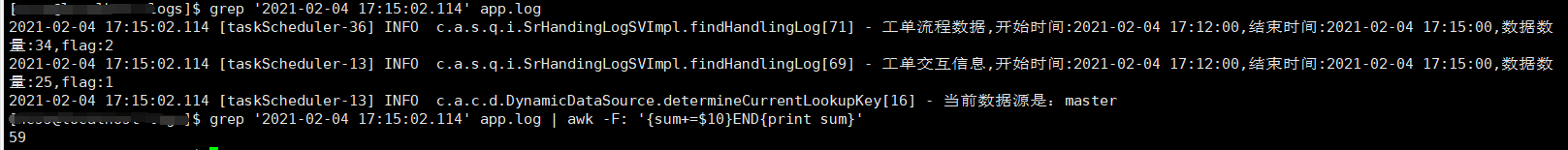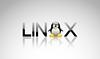• 1、原始文件情况 1.1、文件数 [hadoop@emr-worker-10 result]$ll room54000-htm_2018-* -rw-rw-r-- 1 hadoop hadoop 2 Oct 9 18:59 room54000-...适用于数据跑批后，将每一份的汇总结果再汇总的情况。 1、原始文件情况1.1、文件数 [hadoop@emr-worker-10 result]$ ll room54000-htm_2018-*
-rw-rw-r-- 1 hadoop hadoop 2 Oct  9 19:10 room54000-htm_2018-09-30-16.txt
1.2、文件内容
[hadoop@emr-worker-10 result]$cat room54000-htm_2018-* 0 0 0 0 0 0 0 0 0 0 0 0 0 0 0 0 0 0 7 0 0 0 0 0 2、单列数值统计cat room54000-htm_2018-*|grep -v 0|awk '{sum +=$1};END {print sum}' cat room54000-htm_2018-*|awk '{sum +=$1};END {print sum}' [hadoop@emr-worker-10 result]$ cat room54000-htm_2018-*|awk '{sum +=$1};END {print sum}' 516 [hadoop@emr-worker-10 result]$ cat room54000-htm_2018-*|grep -v 0|awk '{sum +=$1};END {print sum}' 516 [hadoop@emr-worker-10 result]$ 
3、说明适用于数据跑批后，将每一份的汇总结果再汇总的情况。
展开全文awk
• grep ‘查询内容’ app.log | awk -F分列标识 '{sum+=$列数}END{print sum}' 示例： 我这边是将查出来的结果通过":" 分列，然后取的是第10列的数(也就是->) grep ‘查询内容’ app.log | awk -F分列标识 '{sum+=$列数}END{print sum}' 示例：我这边是将查出来的结果通过":" 分列，然后取的是第10列的数(也就是->34,fag和25,flag这两个数) 最后结果是59
ps:awk计算的时候会排除掉非数字字符然后对34、25进行求和
展开全文• 有一个文件，每行都是一个数字，现在需要将每行的数字相加，有如下几种方式。 $cat number_file 1031 2312 2211 42342 1321 3232 213 324 4324 324234 213 34 21 3434 54353 3422242 312 143 方法一：先将换行替换... 有一个文件，每行都是一个数字，现在需要将每行的数字相加，有如下几种方式。 $cat number_file
1031
2312
2211
42342
1321
3232
213
324
4324
324234
213
34
21
3434
54353
3422242
312
143

方法一：先将\n替换为+，然后使用bc命令相加
最开始的想法是直接使用tr命令将\n直接替换为+，但是替换后末尾多了一个+，所以先使用echo -n去掉换行，然后将空格替换为+
第1步：使用echo -n去掉换行
$echo -n cat num_count 1031 2312 2211 42342 1321 3232 213 324 4324 324234 213 34 21 3434 54353 3422242 312 143 第2步：将空格替换为+$echo -n cat num_count | tr ' ' '+'
1031+2312+2211+42342+1321+3232+213+324+4324+324234+213+34+21+3434+54353+3422242+312+143

第3步：使用 bc 命令相加
$echo$(echo -n cat num_count | tr ' ' '+') | bc
3862296

方法二：使用awk
cat num_count |awk '{sum+=$1} END {print sum}' 3862296  展开全文tr bc awk • 通过执行脚本时传递参数的方式，传递7个数值，用while循环在脚本中实现将这7个数值加和??? 有图更好 由于刚学,还不会做,望大神指点指点,赶时间,不然也不会出此下策了,,谢谢了shell 脚本 • LINUX中计算必须用 字符串相加与计算的区别 #!/bin/bash i=0123456789 for a in {1..8} do b=${i:$RANDOM%10:1} ...#结果为数字相加 #!/bin/bash i=0123456789 for a in {1..8} do b=${i:$RANDOM%10:1} ... LINUX中计算必须用 字符串相加与计算的区别 #!/bin/bash i=0123456789 for a in {1..8} do b=${i:$RANDOM%10:1} let p+=$b
done
echo $p #结果为数字相加 #!/bin/bash i=0123456789 for a in {1..8} do b=${i:$RANDOM%10:1} p+=$b
done
echo $p #结果为字符串相加 方法一: expr 1 + 1 expr 2 - 1 expr 2 \* 2 # \转义符号 屏蔽之后一个字符的特殊含义 expr 9 / 3 expr 5 % 2 #求模 取余数 方法二: echo$[1+1]
echo $[8-5] echo$[8*6]
echo $[8/2] echo$[8%3]
方法三:
let   运算结果不显示,通常用于使用运算结果定义变量的值,或者对变量进行自增减
常规写法        主流写法
let a=a+1        let a++
let a=a-1        let a--
let a=a+2        let a+=2
let a=a+b        let a+=b
let a=a*b        let a*=b

方法四:bc计算器,可以计算小数
bc    #常规使用
1+1
2-1
echo 1.1+2 | bc   #非交互式使用
scale3  #定义小数点后长度
echo "scale=3;10/3" |bc  #定义小数点后并运算


展开全文• #设置A变量值 A=aaaaaaaaaa #设置B变量值 B=bbbbbbbbbb #${A}${B} = aaaaaaaaaabbbbbbbbbb #中间可以插入其他字符，如 ${A}@${B}字符拼接
• cmd set /a expression 示例： ...在表达式中的任何非数字字符串键作为环境变量名称，这些环境...Linux num_a= 10 num_b= 11 sum=$(($a + $b )) echo$sum 注：此处有两层括弧， sum=$(($a+$b))cmd • ## Linux实用教程(第三版) 万次阅读 多人点赞 2019-08-27 22:55:59 第一章 Linux系统初步了解 本章内容 1.1 Linux系统简介 1.2 Linux系统的特点和组成 1.3 Linux版本介绍 1.4 Red Hat Linux系统概述 1.1 Linux系统简介 1.1.1 什么是Linux 1.1.2 Linux系统的产生 1.1.3 Linux... • Linux环境下，C语言中整数类型最长的是long long类型，占8个字节，即使如此，其能表示的数仍是有限的。如果想要实现更大的整数相加或其他运算，就不能只用long long类型或者普通整型。我们可以使用字符串来表示整数...c语言 字符串 整数 • linux中一些符号标记：$@ //将所有变量都保存为单独的词  $* //将所有参数保存为一个字符串 ，这两个符号都是可以访问所有变量$# //参数总数  &- //关闭文件  > &2 //重定向到文件描述符，2为文件描述...
• 利用awk命令awk '{print $n}' | awk '{sum+=$1}END{print sum}' 例：awk
• 而对于一个Linux/unix高手来说，这真是小菜一碟，正如文章中介绍的方法，一行命令就完成了这个任务。 (假设文件名叫做data.csv，行数据用竖线分割，我们需要总计其中的第四列。) cat data.csv | awk -F...shell unix 编程
• ## 汇编

千次阅读 2016-12-24 15:42:33
• 1#12@3#2837854155@4#1720@6#18452461013@7#1419923604 截取#号后面的数字然后相加 12+2837854155+1720+18452461013+1419923604awk...

# linux结果相加linux 订阅